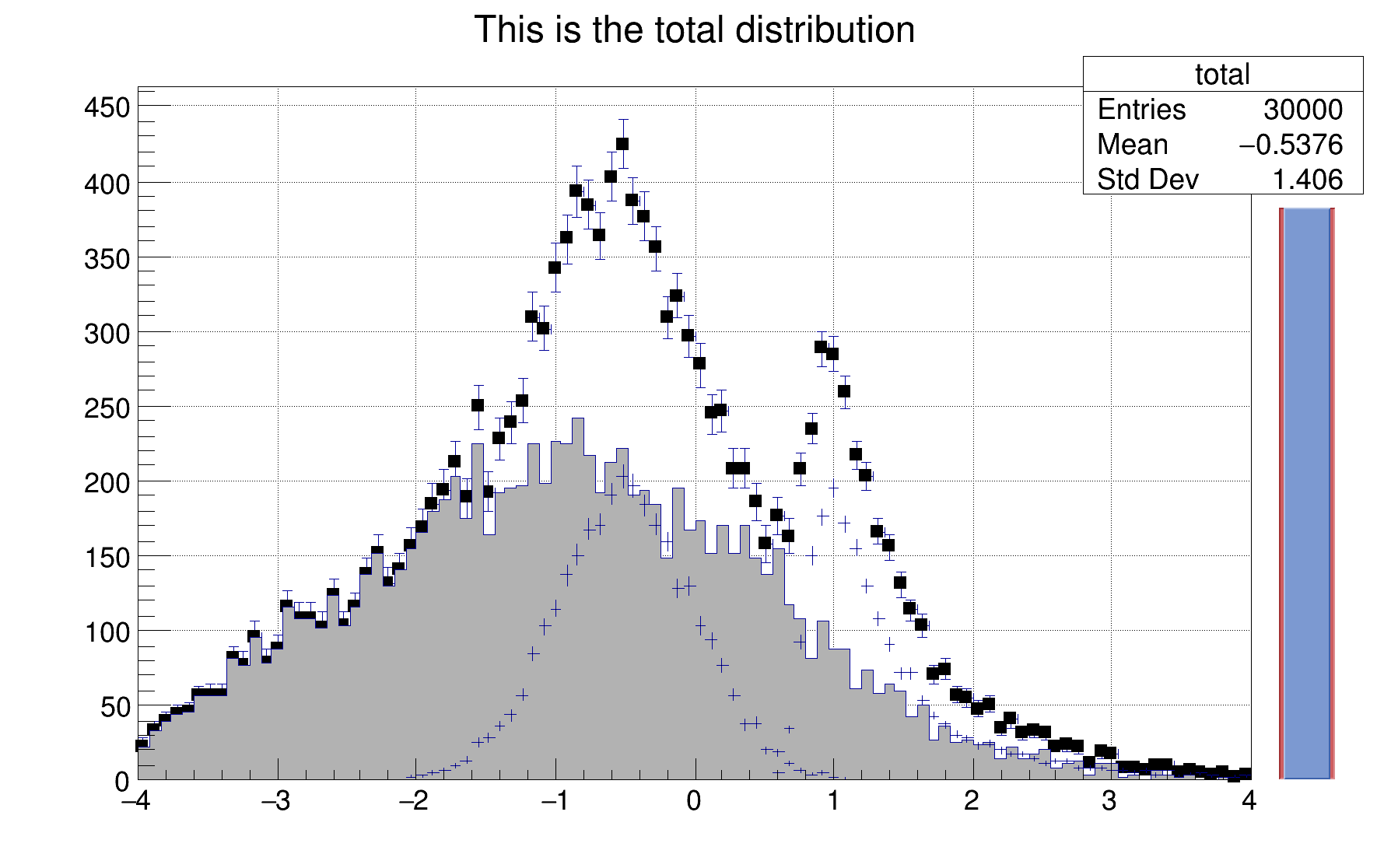ROOT   Reference Guidehsum.C File Reference

## Detailed DescriptionHistograms filled and drawn in a loop.

Simple example illustrating how to use the C++ interpreter to fill histograms in a loop and show the graphics resultsvoid hsum() {
TCanvas *c1 = new TCanvas("c1","The HSUM example",200,10,600,400);
c1->SetGrid();
gBenchmark->Start("hsum");
// Create some histograms.
auto total = new TH1F("total","This is the total distribution",100,-4,4);
auto main = new TH1F("main","Main contributor",100,-4,4);
auto s1 = new TH1F("s1","This is the first signal",100,-4,4);
auto s2 = new TH1F("s2","This is the second signal",100,-4,4);
total->Sumw2(); // store the sum of squares of weights
total->SetMarkerStyle(21);
total->SetMarkerSize(0.7);
main->SetFillColor(16);
s1->SetFillColor(42);
s2->SetFillColor(46);
TSlider *slider = 0;
// Fill histograms randomly
const Int_t kUPDATE = 500;
Float_t xs1, xs2, xmain;
for ( Int_t i=0; i<10000; i++) {
xmain = gRandom->Gaus(-1,1.5);
xs1 = gRandom->Gaus(-0.5,0.5);
xs2 = gRandom->Landau(1,0.15);
main->Fill(xmain);
s1->Fill(xs1,0.3);
s2->Fill(xs2,0.2);
total->Fill(xmain);
total->Fill(xs1,0.3);
total->Fill(xs2,0.2);
if (i && (i%kUPDATE) == 0) {
if (i == kUPDATE) {
total->Draw("e1p");
main->Draw("same");
s1->Draw("same");
s2->Draw("same");
c1->Update();
slider = new TSlider("slider",
"test",4.2,0,4.6,total->GetMaximum(),38);
slider->SetFillColor(46);
}
if (slider) slider->SetRange(0,Float_t(i)/10000.);
c1->Modified();
c1->Update();
}
}
slider->SetRange(0,1);
total->Draw("sameaxis"); // to redraw axis hidden by the fill area
c1->Modified();
gBenchmark->Show("hsum");
}
#define s1(x)
Definition: RSha256.hxx:91
int Int_t
Definition: RtypesCore.h:45
float Float_t
Definition: RtypesCore.h:57
R__EXTERN TBenchmark * gBenchmark
Definition: TBenchmark.h:59
static unsigned int total
R__EXTERN TRandom * gRandom
Definition: TRandom.h:62
virtual void SetFillColor(Color_t fcolor)
Set the fill area color.
Definition: TAttFill.h:37
virtual void Start(const char *name)
Starts Benchmark with the specified name.
Definition: TBenchmark.cxx:172
virtual void Show(const char *name)
Stops Benchmark name and Prints results.
Definition: TBenchmark.cxx:155
The Canvas class.
Definition: TCanvas.h:23
1-D histogram with a float per channel (see TH1 documentation)}
Definition: TH1.h:575
virtual Double_t Gaus(Double_t mean=0, Double_t sigma=1)
Samples a random number from the standard Normal (Gaussian) Distribution with the given mean and sigm...
Definition: TRandom.cxx:274
virtual void SetSeed(ULong_t seed=0)
Set the random generator seed.
Definition: TRandom.cxx:608
virtual Double_t Landau(Double_t mean=0, Double_t sigma=1)
Generate a random number following a Landau distribution with location parameter mu and scale paramet...
Definition: TRandom.cxx:380
A specialized TPad including a TSliderBox object.
Definition: TSlider.h:17
virtual void SetRange(Double_t xmin=0, Double_t xmax=1)
Set Slider range in [0,1].
Definition: TSlider.cxx:207
int main(int argc, char **argv)
return c1
Definition: legend1.C:41
Definition: hsum.py:1

Definition in file hsum.C.